Courses

# Notes | EduRev

## Class 10 : Notes | EduRev

The document Notes | EduRev is a part of the Class 10 Course Mathematics (Maths) Class 10.
All you need of Class 10 at this link: Class 10

Q.9. The angle of elevation of the top of a building from the foot of the tower is 30° and the angle of elevation of the top of the tower from the foot of the building is 60°. If the tower is 50 m high, find the height of the building.
Sol. Given: height of the tower AB = 50 m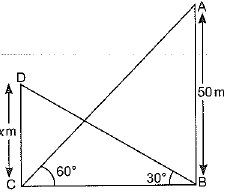∠ACB = 60°, ∠DBC = 30°
Let the height of the building
CD = x m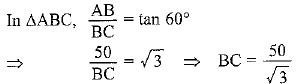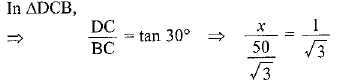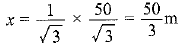Hence, height of the building = 50/3 m

Q.10. Two poles of equal heights are standing opposite each other on either side of the road, which is 80 m wide. From a point between them on the road, the angles of elevation of the top of the poles are 60° and 30° respectively. Find the height of the poles and the distances of the point from the poles.
Sol. Let AB = CD = h m [Height of the poles]
Given: BC = 80 m [Width of the road]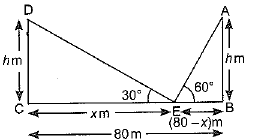Let CE = x m
∴ BE = (80 - x) m
In ΔCDE,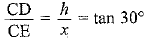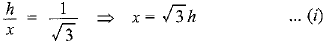In ΔABE,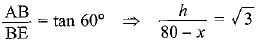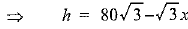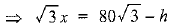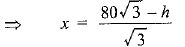... (ii)
From equation (i) and (ii), we get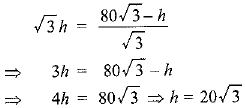Substituting h in equation (i),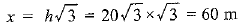Hence, position of the point is at a distance of 60 m from pole CD and 20 m from pole AB.

Q.11. A TV tower stands vertically on a bank of a canal. From a point on the other bank directly opposite the tower, the angle of elevation of the top of the tower is 60°. From another point 20 m away from this point on the line joining this point to the foot of the tower, the angle of elevation of the top of the tower is 30° (see figure). Find the height of the tower and the width of the canal.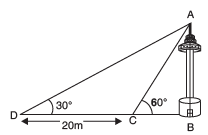Sol. Let the height of the tower AB = h m and BC be the width of the canal.
Given: ∠ACB = 60° and ∠ADB = 30°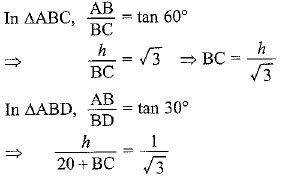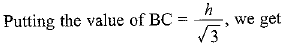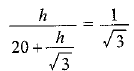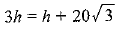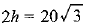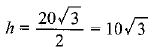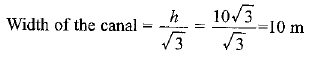Hence, the height of the tower = 10√3 m and width of the canal = 10 m.

Q.12. From the top of a 7 m high building, the angle of elevation of the top of a cable tower is 60° and the angle of depression of its foot is 45°. Determine the height of the tower.
Sol. Let height of the tower AB = (h + 7) m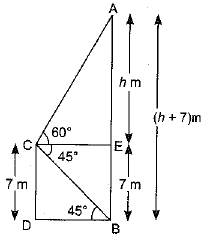Given: CD = 7m (height of the building),
∠ACE = 60°, and ∠ECB = 45°

⇒ ∠CBD = 45º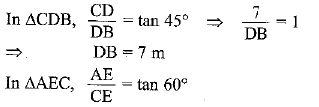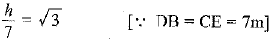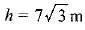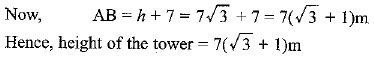Q.13. As observed from the top of a 75 m high lighthouse from the sea-level, the angles of depression of two ships are 30° and 45°. If one ship is exactly behind the other on the same side of the lighthouse, find the distance between the two ships.
Sol. Given: height of the lighthouse = 75 m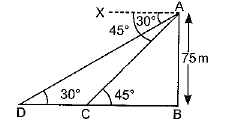Let C and D are the positions of two ships.
We have ∠XAD = ∠ADB = 30°
and ∠XAC = ∠ACB = 45°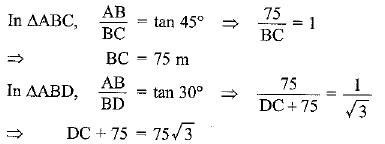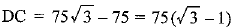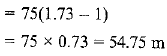Hence, the distance between two ships is 54.75 m.

Q.14. A 1.2 m tall girl spots a balloon moving with the wind in a horizontal line at a height of 88.2 m from the ground. The angle of elevation of the balloon from the eyes of the girl at any instant is 60°. After some time, the angle of elevation reduces to 30° (see figure). Find the distance travelled by the balloon during the interval.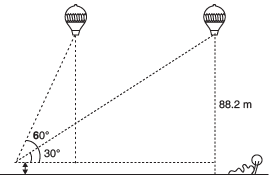Sol. Let the first position of the balloon is A and after some time it will reach to the point D. The vertical height ED = AB = (88.2 - 1.2) m = 87 m.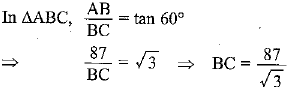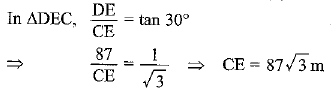Distance travelled by the balloon from A to D is BE.
So, BE = CE - CB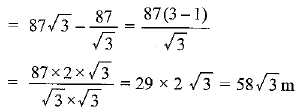Q.15. A straight highway leads to the foot of a tower. A man standing at the top of the tower observes a car at an angle of depression of 30°, which is approaching the foot of the tower with a uniform speed. Six seconds later, the angle of depression of the car is found to be 60°. Find the time taken by the car to reach the foot of the tower from this point.
Sol. Let the height of the tower AB = h m
Given: ∠XAD = ∠ADB = 30°
and ∠XAC - ∠ACB = 60°
Let the speed of the car = x m/sec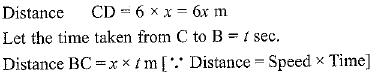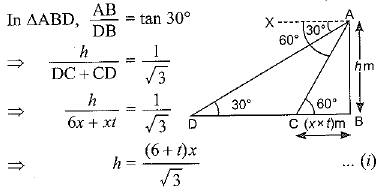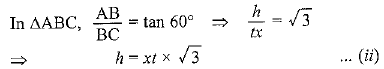From equation (i) and (ii), we get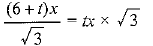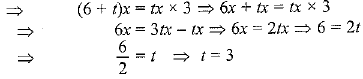Hence, time taken from C to B = 3 sec.

Q.16. The angles of elevation of the top of a tower from two points at a distance of 4 m and 9 m from the base of the tower and in the same straight line with it are complementary. Prove that the height of the tower is 6 m.
Sol. Let the height of the tower AB = h m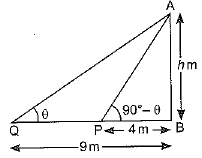We have PB = 4 m, QB = 9 m
Let ∠AQB = θ [Both are complementary angles]
Then ∠APB = 90° - θ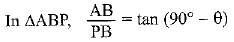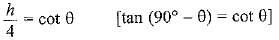⇒  h = 4 cot θ    .......(i)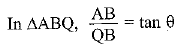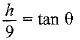h = 9 tan θ    ....(ii)
From equation (i) and (ii), we get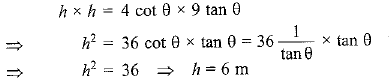Hence, the height of the tower is 6 m.

Offer running on EduRev: Apply code STAYHOME200 to get INR 200 off on our premium plan EduRev Infinity!

## Mathematics (Maths) Class 10

51 videos|346 docs|103 tests

,

,

,

,

,

,

,

,

,

,

,

,

,

,

,

,

,

,

,

,

,

;